### Example 7.7 Simple Pooling Problem

The following optimization problem is discussed in Haverly (1978) and in Liebman et al. (1986, pp. 127–128). Two liquid chemicals,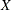and
, are produced by the pooling and blending of three input liquid chemicals,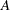,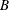, and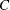. You know the sulfur impurity amounts of the input chemicals, and you have to respect upper limits of the sulfur impurity amounts of the output chemicals. The sulfur concentrations and the prices of the input and output chemicals are:

• Chemical: Concentration = 3%, Price= $6 • Chemical: Concentration = 1%, Price=$16

• Chemical: Concentration = 2%, Price= $10 • Chemical: Concentration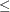2.5%, Price=$9

• Chemical: Concentration1.5%, Price= $15 The problem is complicated by the fact that the two input chemicalsandare available only as a mixture (they are either shipped together or stored together). Because the amounts ofandare unknown, the sulfur concentration of the mixture is also unknown.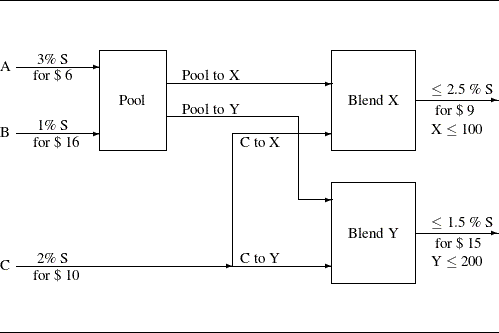You know customers will buy no more than 100 units of X and 200 units of Y. The problem is determining how to operate the pooling and blending of the chemicals to maximize the profit. The objective function for the profit is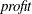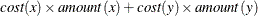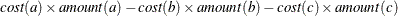There are three groups of constraints: 1. The first group of constraint functions is the mass balance restrictions illustrated by the graph. These are four linear equality constraints: •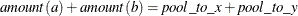•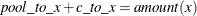•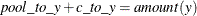•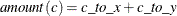2. You introduce a new variable,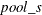, that represents the sulfur concentration of the pool. Usingand the sulfur concentration of(2%), you obtain two nonlinear inequality constraints for the sulfur concentrations ofand, one linear equality constraint for the sulfur balance, and lower and upper boundary restrictions for: •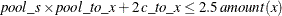•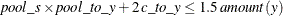•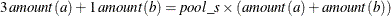•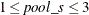3. The last group assembles the remaining boundary constraints. First, you do not want to produce more than you can sell; and finally, all variables must be nonnegative: •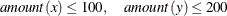•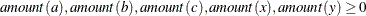•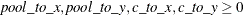There exist several local optima to this problem that can be found by specifying different starting points. Using the starting point with all variables equal to 1 (specified with a PARMS statement), PROC NLP finds a solution with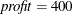: proc nlp all; parms amountx amounty amounta amountb amountc pooltox pooltoy ctox ctoy pools = 1; bounds 0 <= amountx amounty amounta amountb amountc, amountx <= 100, amounty <= 200, 0 <= pooltox pooltoy ctox ctoy, 1 <= pools <= 3; lincon amounta + amountb = pooltox + pooltoy, pooltox + ctox = amountx, pooltoy + ctoy = amounty, ctox + ctoy = amountc; nlincon nlc1-nlc2 >= 0., nlc3 = 0.; max f; costa = 6; costb = 16; costc = 10; costx = 9; costy = 15; f = costx * amountx + costy * amounty - costa * amounta - costb * amountb - costc * amountc; nlc1 = 2.5 * amountx - pools * pooltox - 2. * ctox; nlc2 = 1.5 * amounty - pools * pooltoy - 2. * ctoy; nlc3 = 3 * amounta + amountb - pools * (amounta + amountb); run;  The specified starting point was not feasible with respect to the linear equality constraints; therefore, a starting point is generated that satisfies linear and boundary constraints. Output 7.7.1 gives the starting parameter estimates. Output 7.7.1: Starting Estimates PROC NLP: Nonlinear Maximization Optimization Start Parameter Estimates N Parameter Estimate Gradient Objective Function Gradient Lagrange Function Lower Bound Constraint Upper Bound Constraint 1 amountx 1.363636 9.000000 -0.843698 0 100.000000 2 amounty 1.363636 15.000000 -0.111882 0 200.000000 3 amounta 0.818182 -6.000000 -0.430733 0 . 4 amountb 0.818182 -16.000000 -0.542615 0 . 5 amountc 1.090909 -10.000000 0.017768 0 . 6 pooltox 0.818182 0 -0.669628 0 . 7 pooltoy 0.818182 0 -0.303720 0 . 8 ctox 0.545455 0 -0.174070 0 . 9 ctoy 0.545455 0 0.191838 0 . 10 pools 2.000000 0 0.068372 1.000000 3.000000 Value of Objective Function = 3.8181818182 Value of Lagrange Function = -2.866739915 PROC NLP: Nonlinear Maximization Optimization Results Parameter Estimates N Parameter Estimate Gradient Objective Function Gradient Lagrange Function Active Bound Constraint 1 amountx -1.40474E-11 9.000000 0 Lower BC 2 amounty 200.000000 15.000000 0 Upper BC 3 amounta 1.027701E-16 -6.000000 0 Lower BC 4 amountb 100.000000 -16.000000 -1.77636E-15 5 amountc 100.000000 -10.000000 1.776357E-15 6 pooltox 7.024003E-12 0 0 Lower BC 7 pooltoy 100.000000 0 -1.06581E-14 8 ctox -2.10714E-11 0 5.329071E-15 Lower BC LinDep 9 ctoy 100.000000 0 1.776357E-15 10 pools 1.000000 0 0 Lower BC LinDep The starting point satisfies the four equality constraints, as shown in Output 7.7.2. The nonlinear constraints are given in Output 7.7.3. Output 7.7.2: Linear Constraints PROC NLP: Nonlinear Maximization Linear Constraints 1 -3.331E-16 : ACT 0 == + 1.0000 * amounta + 1.0000 * amountb - 1.0000 * pooltox - 1.0000 * pooltoy 2 1.1102E-16 : ACT 0 == - 1.0000 * amountx + 1.0000 * pooltox + 1.0000 * ctox 3 1.1102E-16 : ACT 0 == - 1.0000 * amounty + 1.0000 * pooltoy + 1.0000 * ctoy 4 1.1102E-16 : ACT 0 == - 1.0000 * amountc + 1.0000 * ctox + 1.0000 * ctoy Output 7.7.3: Nonlinear Constraints PROC NLP: Nonlinear Maximization Values of Nonlinear Constraints Constraint Value Residual Lagrange Multiplier [ 5 ] nlc3 0 0 4.9441 Active NLEC [ 6 ] nlc1_G 0.6818 0.6818 . [ 7 ] nlc2_G -0.6818 -0.6818 -9.8046 Violat. NLIC PROC NLP: Nonlinear Maximization Values of Nonlinear Constraints Constraint Value Residual Lagrange Multiplier [ 5 ] nlc3 0 0 6.0000 Active NLEC [ 6 ] nlc1_G 4.04E-16 4.04E-16 . Active NLIC LinDep [ 7 ] nlc2_G -284E-16 -284E-16 -6.0000 Active NLIC Output 7.7.4 shows the settings of some important PROC NLP options. Output 7.7.4: Options PROC NLP: Nonlinear Maximization Minimum Iterations 0 200 500 20 0.00001 1E-8 0 2.220446E-16 1E-6 0 0 0 0 1.340781E154 2 1 0.4 . 15.653559775 15.653559775 1E-8 1E-8 1E-8 . The iteration history, given in Output 7.7.5, does not show any problems. Output 7.7.5: Iteration History PROC NLP: Nonlinear Maximization Dual Quasi-Newton Optimization Modified VMCWD Algorithm of Powell (1978, 1982) Dual Broyden - Fletcher - Goldfarb - Shanno Update (DBFGS) Lagrange Multiplier Update of Powell(1982) Iteration Restarts Function Calls Objective Function Maximum Constraint Violation Predicted Function Reduction Step Size Maximum Gradient Element of the Lagrange Function 1 0 19 -1.42400 0.00962 6.9131 1.000 0.783 2 ' 0 20 2.77026 0.0166 5.3770 1.000 2.629 3 0 21 7.08706 0.1409 7.1965 1.000 9.452 4 ' 0 22 11.41264 0.0583 15.5769 1.000 23.390 5 ' 0 23 24.84613 8.88E-16 496.1 1.000 147.6 6 0 24 378.22825 147.4 3316.7 1.000 840.4 7 ' 0 25 307.56810 50.9339 607.9 1.000 27.143 8 ' 0 26 347.24468 1.8329 21.9883 1.000 28.482 9 ' 0 27 349.49255 0.00915 7.1833 1.000 28.289 10 ' 0 28 356.58341 0.1083 50.2566 1.000 27.479 11 ' 0 29 388.70731 2.4280 24.7996 1.000 21.114 12 ' 0 30 389.30118 0.0157 10.0475 1.000 18.647 13 ' 0 31 399.19240 0.7997 11.1862 1.000 0.416 14 ' 0 32 400.00000 0.0128 0.1533 1.000 0.00087 15 ' 0 33 400.00000 7.38E-11 2.44E-10 1.000 365E-12 Optimization Results Iterations 15 Function Calls 34 Gradient Calls 18 Active Constraints 10 Objective Function 400 Maximum Constraint Violation 7.381118E-11 Maximum Projected Gradient 0 Value Lagrange Function -400 Maximum Gradient of the Lagran Func 1.065814E-14 Slope of Search Direction -2.43574E-10  FCONV2 convergence criterion satisfied. The optimal solution in Output 7.7.6 shows that to obtain the maximum profit of$400, you need only to produce the maximum 200 units of blendingand no units of blending.

Output 7.7.6: Optimization Solution

PROC NLP: Nonlinear Maximization

Optimization Results
Parameter Estimates
Objective
Function
Lagrange
Function
Active
Bound
Constraint

1 amountx -1.40474E-11 9.000000 0 Lower BC
2 amounty 200.000000 15.000000 0 Upper BC
3 amounta 1.027701E-16 -6.000000 0 Lower BC
4 amountb 100.000000 -16.000000 -1.77636E-15
5 amountc 100.000000 -10.000000 1.776357E-15
6 pooltox 7.024003E-12 0 0 Lower BC
7 pooltoy 100.000000 0 -1.06581E-14
8 ctox -2.10714E-11 0 5.329071E-15 Lower BC LinDep
9 ctoy 100.000000 0 1.776357E-15
10 pools 1.000000 0 0 Lower BC LinDep

Value of Objective Function = 400

Value of Lagrange Function = 400

The constraints are satisfied at the solution, as shown in Output 7.7.7

Output 7.7.7: Linear and Nonlinear Constraints at the Solution

PROC NLP: Nonlinear Maximization

Linear Constraints Evaluated at Solution
1 ACT 0 = 0 + 1.0000 * amounta + 1.0000 * amountb - 1.0000 * pooltox - 1.0000 * pooltoy
2 ACT -4.481E-17 = 0 - 1.0000 * amountx + 1.0000 * pooltox + 1.0000 * ctox
3 ACT 0 = 0 - 1.0000 * amounty + 1.0000 * pooltoy + 1.0000 * ctoy
4 ACT 0 = 0 - 1.0000 * amountc + 1.0000 * ctox + 1.0000 * ctoy

Values of Nonlinear Constraints
Constraint Value Residual Lagrange
Multiplier

[ 5 ] nlc3 0 0 6.0000 Active NLEC
[ 6 ] nlc1_G 4.04E-16 4.04E-16 . Active NLIC LinDep
[ 7 ] nlc2_G -284E-16 -284E-16 -6.0000 Active NLIC

Linearly Dependent Active Boundary
Constraints
Parameter N Kind
ctox 8 Lower BC
pools 10 Lower BC

Linearly Dependent
Nonlinear Constraints
Parameter N
nlc3 6

The same problem can be specified in many different ways. For example, the following specification uses an INEST= data set containing the values of the starting point and of the constants COST, COSTB, COSTC, COSTX, COSTY, CA, CB, CC, and CD:

data init1(type=est);
input _type_ $amountx amounty amounta amountb amountc pooltox pooltoy ctox ctoy pools _rhs_ costa costb costc costx costy ca cb cc cd; datalines; parms 1 1 1 1 1 1 1 1 1 1 . 6 16 10 9 15 2.5 1.5 2. 3. ;  proc nlp inest=init1 all; parms amountx amounty amounta amountb amountc pooltox pooltoy ctox ctoy pools; bounds 0 <= amountx amounty amounta amountb amountc, amountx <= 100, amounty <= 200, 0 <= pooltox pooltoy ctox ctoy, 1 <= pools <= 3; lincon amounta + amountb = pooltox + pooltoy, pooltox + ctox = amountx, pooltoy + ctoy = amounty, ctox + ctoy = amountc; nlincon nlc1-nlc2 >= 0., nlc3 = 0.; max f; f = costx * amountx + costy * amounty - costa * amounta - costb * amountb - costc * amountc; nlc1 = ca * amountx - pools * pooltox - cc * ctox; nlc2 = cb * amounty - pools * pooltoy - cc * ctoy; nlc3 = cd * amounta + amountb - pools * (amounta + amountb); run;  The third specification uses an INEST= data set containing the boundary and linear constraints in addition to the values of the starting point and of the constants. This specification also writes the model specification into an OUTMOD= data set: data init2(type=est); input _type_$ amountx amounty amounta amountb amountc
pooltox pooltoy ctox ctoy pools
_rhs_ costa costb costc costx costy;
datalines;
parms      1   1  1  1  1    1   1   1  1  1
.   6 16 10  9   15 2.5 1.5  2  3
lowerbd    0   0  0  0  0    0   0   0  0  1
.   .  .  .  .    .   .   .  .  .
upperbd  100 200  .  .  .    .   .   .  .  3
.   .  .  .  .    .   .   .  .  .
eq         .   .  1  1  .   -1  -1   .  .  .
0   .  .  .  .    .   .   .  .  .
eq         1   .  .  .  .   -1   .  -1  .  .
0   .  .  .  .    .   .   .  .  .
eq         .   1  .  .  .    .  -1   . -1  .
0   .  .  .  .    .   .   .  .  .
eq         .   .  .  .  1    .   .  -1 -1  .
0   .  .  .  .    .   .   .  .  .
;

proc nlp inest=init2 outmod=model all;
parms amountx amounty amounta amountb amountc
pooltox pooltoy ctox ctoy pools;
nlincon nlc1-nlc2 >= 0.,
nlc3 = 0.;
max f;
f = costx * amountx + costy * amounty
- costa * amounta - costb * amountb - costc * amountc;
nlc1 = 2.5 * amountx - pools * pooltox - 2. * ctox;
nlc2 = 1.5 * amounty - pools * pooltoy - 2. * ctoy;
nlc3 = 3 * amounta + amountb - pools * (amounta + amountb);
run;


The fourth specification not only reads the INEST=INIT2 data set, it also uses the model specification from the MODEL data set that was generated in the last specification. The PROC NLP call now contains only the defining variable statements:

proc nlp inest=init2 model=model all;
parms amountx amounty amounta amountb amountc
pooltox pooltoy ctox ctoy pools;
nlincon nlc1-nlc2 >= 0.,
nlc3 = 0.;
max f;
run;


All four specifications start with the same starting point of all variables equal to 1 and generate the same results. However, there exist several local optima to this problem, as is pointed out in Liebman et al. (1986, p. 130).

proc nlp inest=init2 model=model all;
parms amountx amounty amounta amountb amountc
pooltox pooltoy ctox ctoy = 0,
pools = 2;
nlincon nlc1-nlc2 >= 0.,
nlc3 = 0.;
max f;
run;


This starting point with all variables equal to 0 is accepted as a local solution with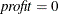, which minimizes rather than maximizes the profit.